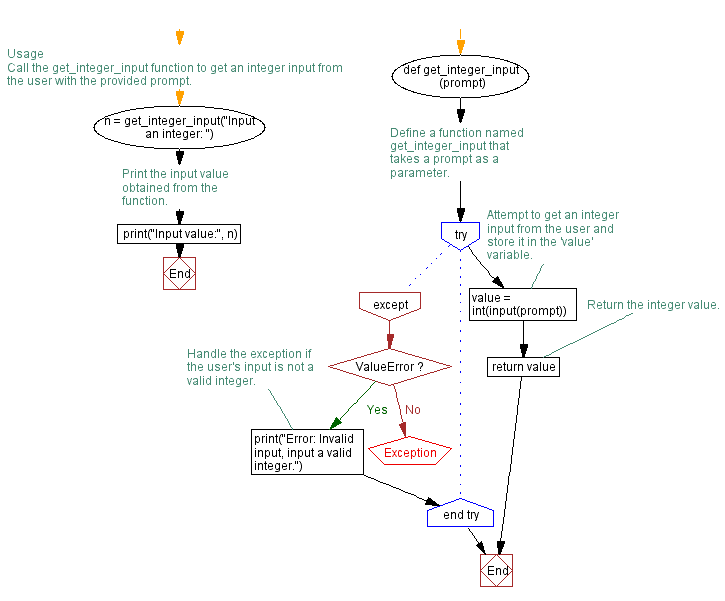﻿ Python Program for valid integer input

# Handling ValueError Exception in Python integer input program

## Python Exception Handling: Exercise-2 with Solution

Write a Python program that prompts the user to input an integer and raises a ValueError exception if the input is not a valid integer.

exception ValueError:

Raised when an operation or function receives an argument that has the right type but an inappropriate value, and the situation is not described by a more precise exception such as IndexError.

Sample Solution:

Code:

``````# Define a function named get_integer_input that takes a prompt as a parameter.
def get_integer_input(prompt):
try:
# Attempt to get an integer input from the user and store it in the 'value' variable.
value = int(input(prompt))
# Return the integer value.
return value
except ValueError:
# Handle the exception if the user's input is not a valid integer.
print("Error: Invalid input, input a valid integer.")

# Usage
# Call the get_integer_input function to get an integer input from the user with the provided prompt.
n = get_integer_input("Input an integer: ")
# Print the input value obtained from the function.
print("Input value:", n)
``````

Explanation:

In the above code, the get_integer_input function takes a prompt parameter that represents the message displayed to the user for input.

Within the try block, we use the int() function to convert user input to an integer. If the conversion succeeds, we return the integer value.

If the conversion fails and a ValueError exception is raised, the program jumps to the except block. In the except block, we catch the ValueError exception and print an error message indicating the input is invalid.

Output:

```Input an integer: abc
Error: Invalid input, input a valid integer.
Input value: None
```
```Input an integer: 10.06
Error: Invalid input, input a valid integer.
Input value: None
```
```Input an integer: 5
Input value: 5
```

Flowchart:What is the difficulty level of this exercise?

Test your Programming skills with w3resource's quiz.

﻿